# Research Projects

• ## Searching for combinatorial specifications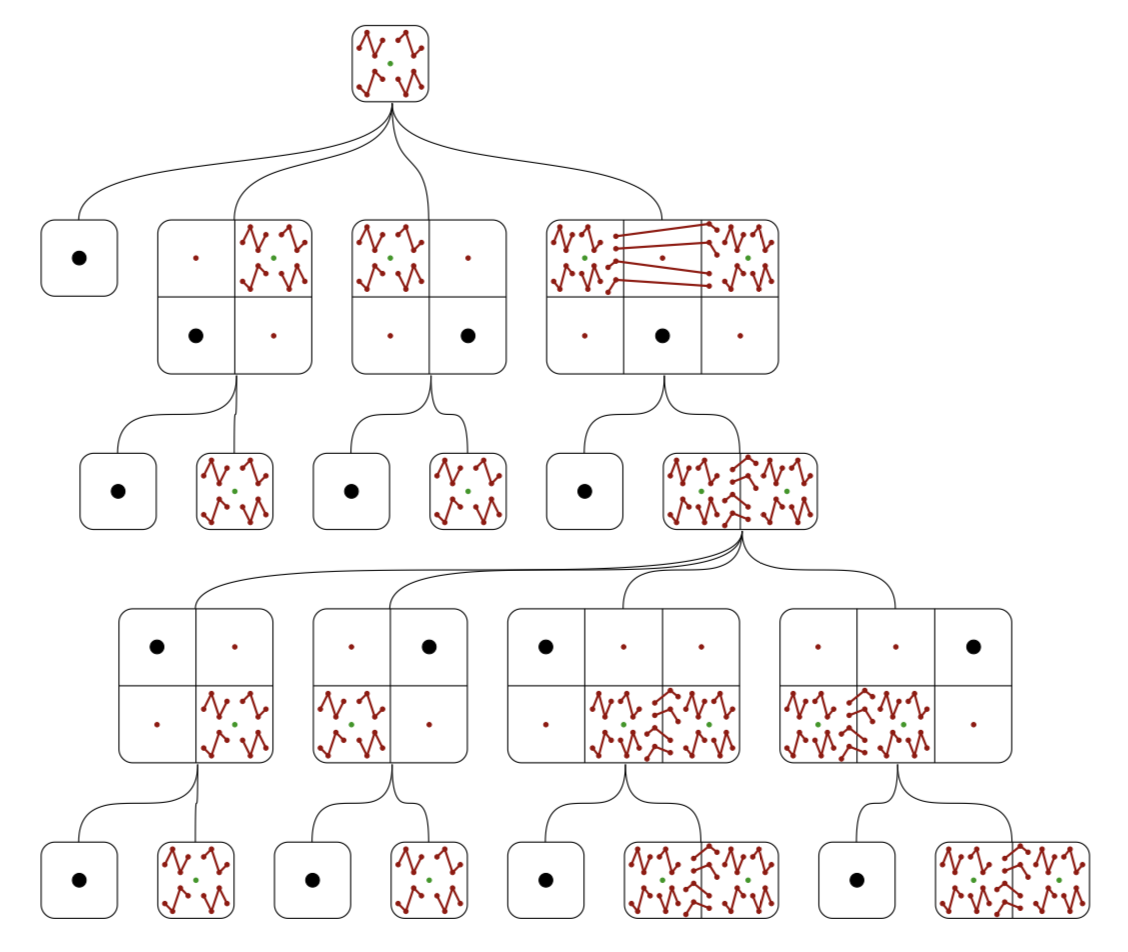A combinatorial class is often studied by finding a combinatorial specification for it, which is a description of the class in terms of simpler classes, and perhaps recursions to smaller instances of the original class. This project aims to automate the discovery of combinatorial specifications of combinatorial classes. This is achieved by first generating a universe of interconnected combinatorial classes and then searching for a specification inside this universe. The main application has been in the area of permutation patterns, but other domains such as, set partitions, strings, polyominoes and lattice paths have been considered.

• ## Automatic discovery of conjectured covers of combinatorial classes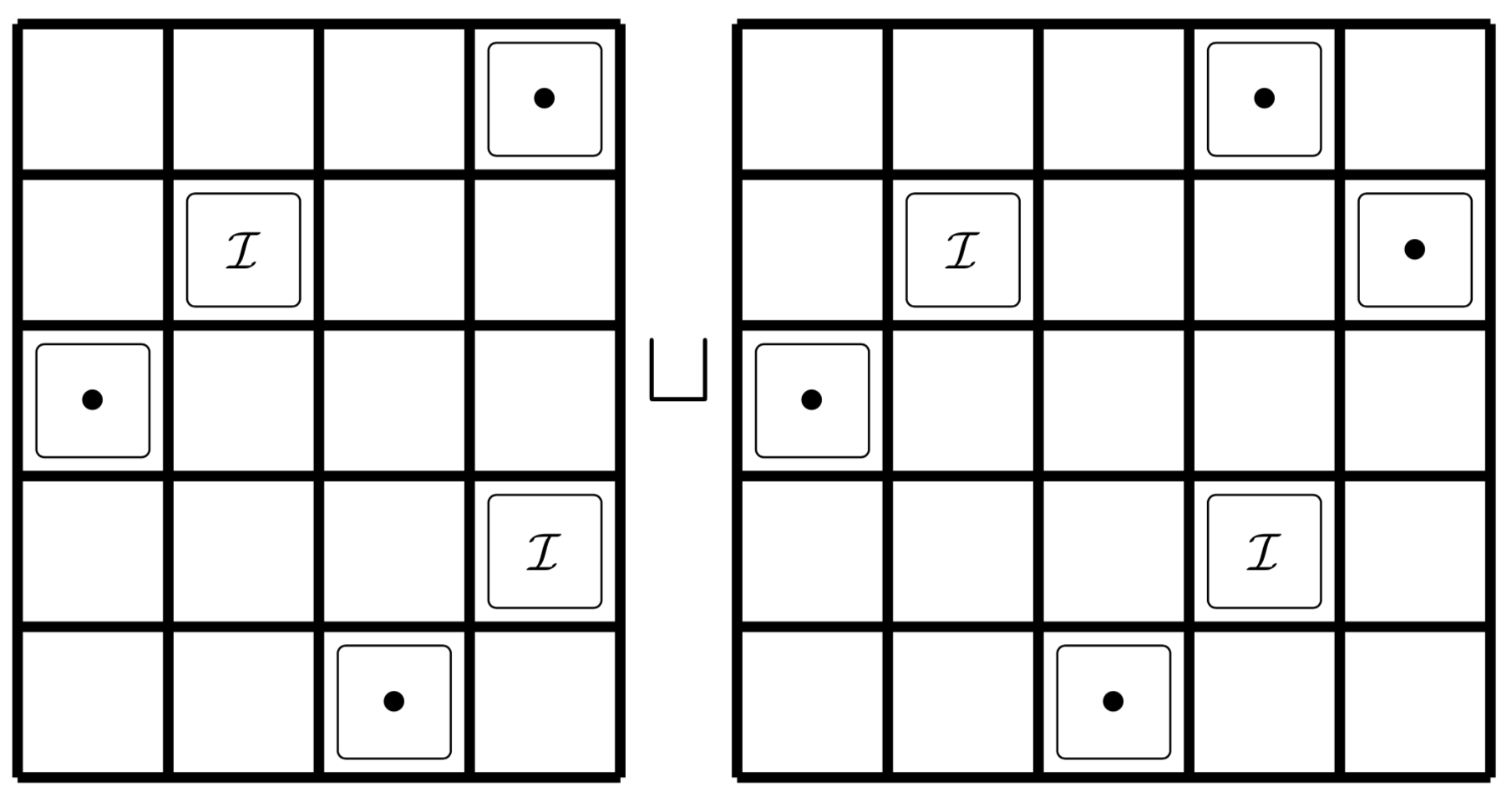This project started with an IRF grant in 2014 and the hiring of Christian as a PhD student. The idea is to guess an exact cover a combinatorial class. Currently we have a program that does this in the case of a permutation class.

• ## Sorting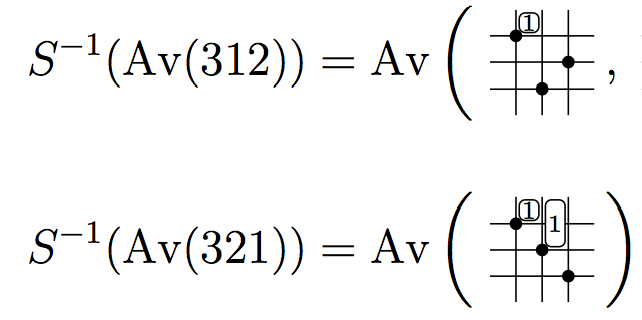We introduce an algorithm to determine when a sorting operation, such as stack-sort or bubble-sort, outputs a given pattern. The algorithm provides a new proof of the description of West-2-stack-sortable permutations, that is permutations that are completely sorted when passed twice through a stack, in terms of patterns. We also solve the long-standing problem of describing West-3-stack-sortable permutations. This requires a new type of generalized permutation pattern we call a decorated pattern.

• ## Heuristic algorithms for pattern and statistics discovery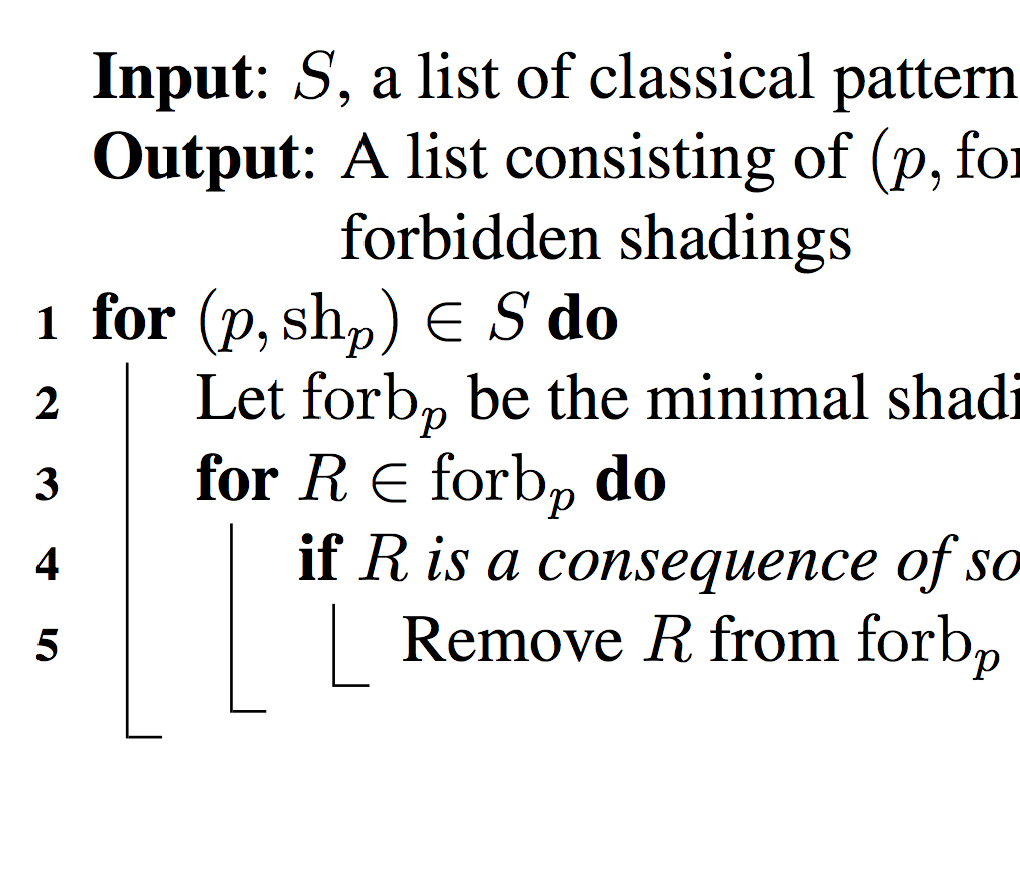We study algorithms that given a finite set of permutations conjecture (1) a basis of (mesh) patterns describing the set; (2) a structural description of the set, that can then be translated into a generating function enumerating the set.

• ## Coincidence and Wilf-classification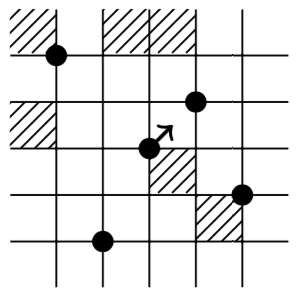Two patterns are coincident if they are avoided by the same permutations. They are Wilf-equivalent if the are avoided by the same number of permutations. We study general rules which can tell us when patterns (or sets of patterns) are coincident or Wilf-equivalent.

• ## Schubert varieties and permutation patternsDescription.